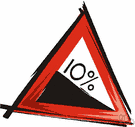Also found in: Thesaurus, Medical, Financial, Encyclopedia.

## gra·di·ent

(grā′dē-ənt)
1. A rate of inclination; a slope.
2. An ascending or descending part; an incline.
3. Physics The rate at which a physical quantity, such as temperature or pressure, changes in response to changes in a given variable, especially distance.
4. Mathematics A vector having coordinate components that are the partial derivatives of a function with respect to its variables.
5. Biology A series of progressively increasing or decreasing differences in the growth rate, metabolism, or physiological activity of a cell, organ, or organism.

[Perhaps grade + -ient (as in quotient).]

(ˈɡreɪdɪənt)
n
1. (Civil Engineering) Also called (esp US): grade a part of a railway, road, etc, that slopes upwards or downwards; inclination
2. (Civil Engineering) Also called (esp US and Canadian): grade a measure of such a slope, esp the ratio of the vertical distance between two points on the slope to the horizontal distance between them
3. (General Physics) physics a measure of the change of some physical quantity, such as temperature or electric potential, over a specified distance
4. (Mathematics) maths
a. (of a curve) the slope of the tangent at any point on a curve with respect to the horizontal axis
b. (of a function, f(x, y, z)) the vector whose components along the axes are the partial derivatives of the function with respect to each variable, and whose direction is that in which the derivative of the function has its maximum value. Usually written: grad f, ∇f or ∇f. Compare curl11, divergence4
sloping uniformly

## gra•di•ent

(ˈgreɪ di ənt)

n.
1. the degree of inclination of a highway, railroad, etc., or the rate of ascent or descent of a stream or river.
2. an inclined surface; grade; ramp.
3.
a. the rate of change with respect to distance of a variable quantity, as temperature or pressure, in the direction of maximum change.
b. a curve representing such a rate of change.
4. a differential operator that, operating upon a function of several variables, results in a vector whose coordinates are the partial derivatives of the function. Abbr.: grad. Symbol:
5. rising or descending by regular degrees of inclination.
6. progressing by walking; stepping with the feet as animals do.
Closely spaced contour lines on the right indicate a steeper gradient than the more loosely spaced lines on the left.

## gra·di·ent

(grā′dē-ənt)
1. The degree to which something inclines; a slope. A mountain road with a gradient of ten percent rises one foot for every ten feet of horizontal length.
2. The rate at which a physical quantity, such as temperature or pressure changes over a distance.

The rate of inclination to horizontal expressed as a ratio, such as 1:25, indicating a one unit rise to 25 units of horizontal distance.
ThesaurusAntonymsRelated WordsSynonymsLegend:
 Noun 1gradient - a graded change in the magnitude of some physical quantity or dimensionchange - a relational difference between states; especially between states before and after some event; "he attributed the change to their marriage"concentration gradient - a gradient in concentration of a solute as a function of distance through a solution; "the movement of a solute down its concentration gradient is called diffusion"gravity gradient - a gradient in the gravitational forces acting on different parts of a nonspherical object; "the gravity gradient of the moon causes the ocean tides on Earth"temperature gradient - change in temperature as a function of distance (especially altitude) 2 gradient - the property possessed by a line or surface that departs from the horizontal; "a five-degree gradient"slopegrade - the gradient of a slope or road or other surface; "the road had a steep grade"rake, slant, pitch - degree of deviation from a horizontal plane; "the roof had a steep pitch"precipitousness, steepness, abruptness - the property possessed by a slope that is very steepgentleness, gradualness - the property possessed by a slope that is very gradualspatial relation, position - the spatial property of a place where or way in which something is situated; "the position of the hands on the clock"; "he specified the spatial relations of every piece of furniture on the stage"

noun a hill with a gradient of 1 in 3

noun
Deviation from a particular direction:
Translations
درجَة المَيْل أو الأنْحِدارمُنْحَدَر
sklonstupeň sklonu
lejtõsség
hallihalli, hallagráîa, stigull
nogāzeslīpums
stupeň sklonu

[ˈgreɪdɪənt] N (esp Brit) →
a gradient of one in sevenuna pendiente del uno por siete

[ˈgreɪdiənt] n
(= slope) →
(GEOMETRY)

n (esp Brit) → Neigung f; (upward also) → Steigung f; (downward also) → Gefälle nt; a gradient of 1 in 10eine Steigung/ein Gefälle von 10%; what is the gradient?wie groß ist die Steigung/das Gefälle?; what is the gradient of the hill?welche Steigung/welches Gefälle hat der Berg?

[ˈgreɪdɪənt] n
a gradient of 1 in 7 → una pendenza del 7 per cento
b. (Math, Phys) →

(ˈgreidiənt) noun
1. the amount of slope (eg of a road, a railway). a gradient of 1 in 4.
2. a slope.

## gra·di·ent

a. gradiente, línea que indica aumento o disminución en una variable.
References in periodicals archive ?
For one input images G, on the one hand, we want the target image U to be as close as possible to G, while U should be as smooth as possible except for some places where the edge gradient changes a lot in G.
These markers correspond to the background of the image, combine the foreground markers with the background markers to reconstruct the edge gradient topographic map, and get the smooth cell boundary through the second watershed.
We use multiobjective optimization method (maximizing the difference between target and background, improving the uniformity within the target region, and considering the edge gradient) to improve the segmentation performance.

Site: Follow: Share:
Open / Close